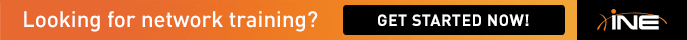# IEWBRS VOl1 v5:QOS:Task2:MTU value?

hi,

how did you guys calculated the MTU value?

The fragement size is calculated as: Fragment Size = Bandwidth x Delay

here: BW=128kbps (assuming the line-rate) and Delay=10ms

=> Fragment=128000 x (10/1000)=1280 bits

now 8-bits = 1-byte

=> 1280-bits = 1280/8 = 160 bytes

Please explain????

Regards,
Khalid

Sign In or Register to comment.